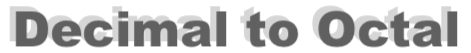Decimal to Octal ConverterWelcome to the Decimal to Octal Converter. Here you submit a decimal number and we will show you detailed step-by-step instructions on how to convert your decimal number to an octal number.

Use this converter to become a decimal to octal converter expert!
To begin, please enter your decimal number below and press "To Octal".

Here are some examples of what our Decimal to Octal Converter can answer in great detail:

How to convert 9 to octal

How to convert 16 to octal

How to convert 59 to octal

How to convert 93 to octal

How to convert 596 to octal

How to convert 1472 to octal